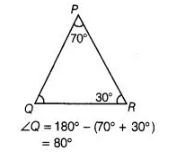# In ΔPQR, ∠P = 70° and ∠R = 30°.`
Question:

In ΔPQR, ∠P = 70° and ∠R = 30°. Which side of this triangle is the longest? Give reason for your answer.

Solution:

Given, in ΔPQR, ∠P = 70° and ∠R = 30°.

We know that, sum of all the angles of a triangle is 180°.

∠P + ∠Q + ∠R = 180°We know that here ∠Q is longest, so side PR is longest.

[∴ since in a triangle, the side opposite to the largest angle is the longest]